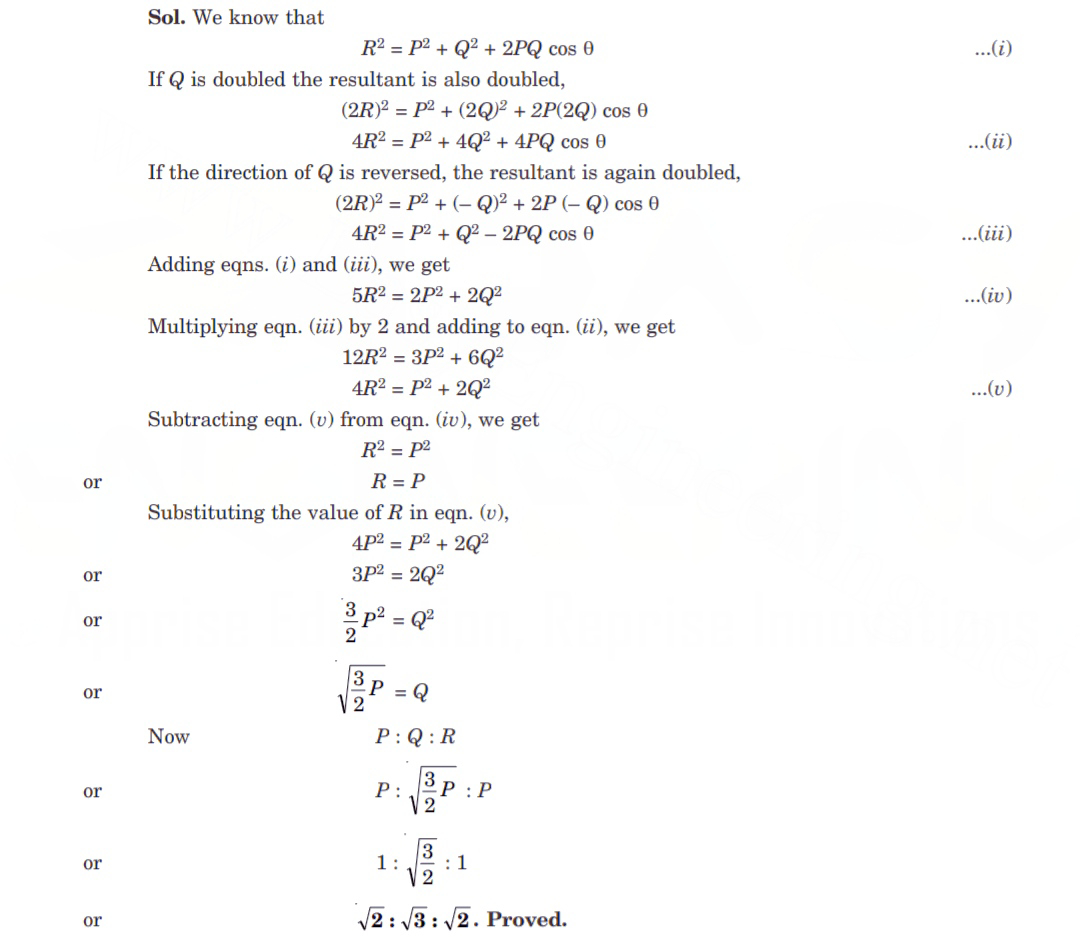# Two forces P and Q acting at a point have a resultant R. If Q be doubled, R is doubled. Again if the direction of Q is reversed, then R is doubled, show that P : Q : R = √2:√3:√2.

Question-AnswerTwo forces P and Q acting at a point have a resultant R. If Q be doubled, R is doubled. Again if the direction of Q is reversed, then R is doubled, show that P : Q : R = √2:√3:√2.

### Two forces P and Q acting at a point have a resultant R. If Q be doubled, R is doubled. Again if the direction of Q is reversed, then R is doubled, show that P : Q : R = √2:√3:√2.